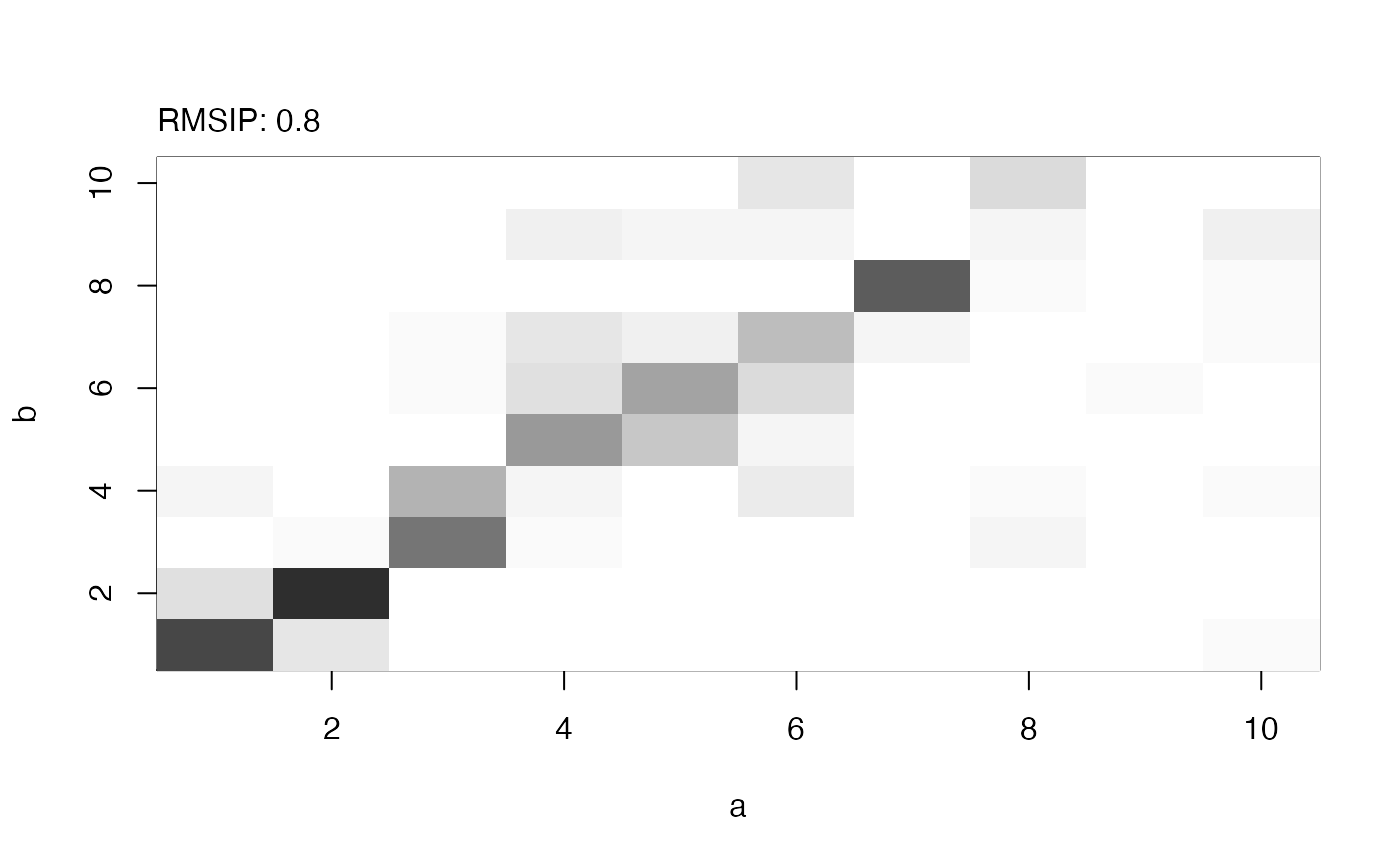Produces a heat plot of RMSIP (Root mean square inner product) for the visualization of modes similarity.

# S3 method for rmsip
plot(x, xlab = NULL, ylab = NULL, col = gray(50:0/50),
zlim=c(0,1), ...)

## Arguments

x an object of class rmsip. a label for the x axis, defaults to ‘a’. a label for the y axis, defaults to ‘b’. a vector of colors for the RMSIP map (or overlap values). the minimum and maximum ‘z’ values for which colors should be plotted. additional arguments to function image.

## Details

plot.rmsip produces a color image with the function image.

## Value

Called for its effect.

Grant, B.J. et al. (2006) Bioinformatics 22, 2695--2696.

## Author

Lars Skjaerven

rmsip, overlap, nma, image.

## Examples

## Read PDB structure
pdb <- read.pdb( system.file("examples/1hel.pdb", package="bio3d") )

## Perform NMA
modes.a <- nma(pdb, ff="calpha")
#>  Building Hessian...		Done in 0.015 seconds.
#>  Diagonalizing Hessian...	Done in 0.086 seconds.modes.b <- nma(pdb, ff="anm")
#>  Building Hessian...		Done in 0.021 seconds.
#>  Diagonalizing Hessian...	Done in 0.087 seconds.
## Calculate and plot RMSIP
r <- rmsip(modes.a, modes.b)
plot(r)